#### Howdy, Stranger!

It looks like you're new here. If you want to get involved, click one of these buttons!

Options

# Lecture 45 - Chapter 3: Composing Natural Transformations

edited February 18

I told you about categories. I told you about functors. Now I'm going to tell you about functor categories!

I remember how this kind of thing used to make me panic. I had learned a couple of concepts in category theory, and I had them neatly separate in my mind - but then someone combined them in a way that was new to me, and my brain short-circuited. Eventually I realized these combinations are exactly what makes category theory so powerful, and I grew to enjoy them.

But in this particular case there's really no need to panic. You may know the idea of a set of functions, like the set of all functions from a set $$X$$ to a set $$Y$$. This is called $$Y^X$$. That may look backwards, but there's a good reason: there are $$3^7$$ functions from a $$7$$-element to a $$3$$-element set.

Just as you can form a set of functions, you can form a category of functors. There's a category of all functors from a category $$\mathcal{C}$$ to a category $$\mathcal{D}$$. It's called $$\mathcal{D}^\mathcal{C}$$.

How does it work? The objects of $$\mathcal{D}^\mathcal{C}$$ are just functors from $$\mathcal{C}$$ to a category $$\mathcal{D}$$. That's the easy part. But what are the morphisms?

The morphisms are natural transformations!

You should have seen that coming. When we have two functors $$F,G : \mathcal{C} \to \mathcal{D}$$, we can talk about natural transformations from $$F$$ to $$G$$. We write these using double arrows, like $$\alpha: F \Rightarrow G$$, just so they look different from functors. And now I'm just saying we should take these double arrows seriously: a natural transformation $$\alpha: F \Rightarrow G$$ is a kind of morphism from $$F$$ to $$G$$.

But the big question is: how do we compose these guys?

Here's how:

Definition. Let $$\mathcal{C}$$ and $$\mathcal{D}$$ be categories, let $$F,G,H : \mathcal{C} \to \mathcal{D}$$ be functors, and let $$\alpha : F \Rightarrow G$$ and $$\beta : G \Rightarrow H$$ be natural transformations. Then there is a natural transformation $$\beta \circ \alpha : F \Rightarrow H$$, called the composite of $$\alpha$$ and $$\beta$$, defined as follows:

$$(\beta \circ \alpha)_x = \beta_x \circ \alpha_x$$ for any object $$x \in \mathcal{C}$$.

This is a reasonable definition. After all,

$$\alpha_x : F(x) \to G(x)$$ and

$$\beta_x : G(x) \to H(x)$$ so they are just dying to be composed to give

$$\beta_x \circ \alpha_x : F(x) \to H(x) .$$ But we still need to check that this gives a natural transformation! Remember, for a transformation to be natural we need a bunch of 'naturality squares' to commute.

Let's check this out. Since $$\alpha$$ is natural we know that for any morphism $$f: x \to y$$ in $$\mathcal{C}$$ this square commutes: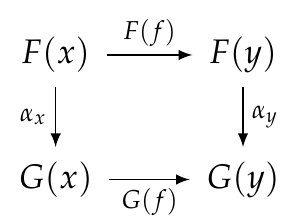Remember, that just means that going down and then across is equal to going across and then down:

$$G(f) \circ \alpha_x = \alpha_y \circ F(f) .$$ Similarly, since $$\beta$$ is natural we know that this square commutes: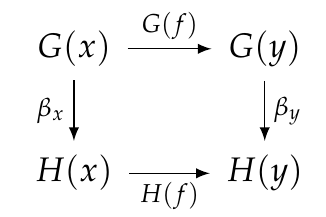or in other words, this equation holds:

$$H(f) \circ \beta_x = \beta_y \circ G(f) .$$ We need to show that $$\beta \circ \alpha$$ is natural, meaning that this square commutes: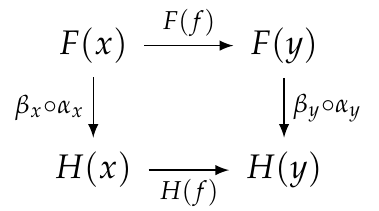or in other words

$$H(f) \circ \beta_x \circ \alpha_x = \beta_y \alpha_y F(f) .$$ We can prove this equation using the previous two, like this:

$$H(f) \circ \beta_x \circ \alpha_x = \beta_y \circ G(f) \circ \alpha_x = \beta_y \circ \alpha_y \circ F(f) .$$ Yay!

Please make sure you follow this simple calculation perfectly. Don't gloss over it, really think about it! Here's another way to think about it. We can take the naturality squares for $$\alpha$$ and $$\beta$$ and stick them together, like this: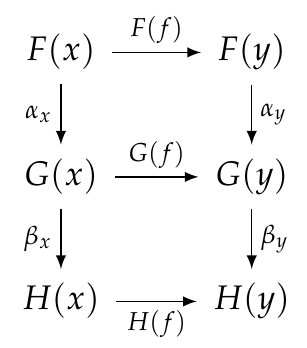For each square, down and then across = across and then down. So, this is true for the whole big rectangle! That gives us the naturality square we need:This kind of reasoning with diagrams turns out to be easier than messing with equations. When you get good at it, you just draw a bunch of squares and triangles and other shapes and stare at them and see that what you want is true! It's called 'diagram chasing'.

So, we can compose two natural transformations and get another natural transformations. We also have 'identity' natural transformations:

Definition. For any functor $$F : \mathcal{C} \to \mathcal{D}$$ there is an identity natural transformation $$1_F : F \Rightarrow F$$, with

$$(1_F)_x = 1_x$$ for all $$x \in \mathcal{C}$$.

So, we're well on our way to getting a category where objects are functors from $$\mathcal{C}$$ to $$\mathcal{D}$$ and morphisms are natural transformations between such functors! But we've got to check some stuff:

Puzzle 140. Show that $$1_F : F \Rightarrow F$$, defined this way, really is natural.

Puzzle 141. Prove the left and right unit laws: for any natural transformation $$\alpha: F \Rightarrow G$$ we have

$$1_G \circ \alpha = \alpha = \alpha \circ 1_F .$$ Puzzle 142. Prove the associative law: for any natural transformations $$\alpha: F \Rightarrow G$$, $$\beta: G \Rightarrow H$$ and $$\gamma: H \Rightarrow I$$ we have

$$(\gamma \circ \beta) \circ \alpha = \gamma \circ (\beta \circ \alpha).$$ That's all we need to check! So now we're ready to announce our result and celebrate!

Theorem. For any categories $$\mathcal{C}$$ and $$\mathcal{D}$$ there is a functor category $$\mathcal{D}^\mathcal{C}$$ where:

1. objects are functors from $$\mathcal{C}$$ to $$\mathcal{D}$$,

2. morphisms are natural transformations between such functors,

3. composition and identities are defined as above.

Great!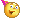What next? Well, you should think about how this relates to databases:

Puzzle 143. Make up an example of three databases built using the same schema, say $$F,G,H : \mathcal{C} \to \mathbf{Set}$$. Make up some natural transformations $$\alpha : F \Rightarrow G$$ and $$\beta : G \Rightarrow H$$, and figure out what happens when you compose them.

But the fun with databases will really start when we understand adjoint functors and 'Kan extensions'. Everything I've done for the last few days is leading up to that material. It's some of the most important, fundamental category theory there is!

To read other lectures go here.

• Options
1.

Puzzle 140. Show that $$1_F:F⇒F$$, defined this way, really is natural.

Each naturality square is of the form $$F_{A\to B}(f) \circ id_{F(A)} = id_{F(B)} \circ F_{A\to B}(f)$$ which commutes because all $$id_{*}$$ are left and right units. QED

This was weirdly hard for me to write out a proof of, I ?knew? that it was natural because id "doesn't do anything" but figuring out what that actually means formally seemed hard.

Comment Source:**Puzzle 140.** Show that \$$1_F:F⇒F\$$, defined this way, really is natural. Each naturality square is of the form \$$F_{A\to B}(f) \circ id_{F(A)} = id_{F(B)} \circ F_{A\to B}(f)\$$ which commutes because all \$$id_{*} \$$ are left and right units. **QED** This was weirdly hard for me to write out a proof of, I ?knew? that it was natural because id "doesn't do anything" but figuring out what that actually means formally seemed hard.
• Options
2.
edited June 2018

Puzzle 141:
Because $$G(f) \circ 1_{G(Y)}=G(f)$$ and $$1_{G(X)} \circ G(f)=G(f)$$ we have $$1_G \circ \alpha =\alpha$$
Same for $$\alpha=\alpha \circ 1_F$$

Comment Source:<b>Puzzle 141:</b><br> Because \$$G(f) \circ 1_{G(Y)}=G(f)\$$ and \$$1_{G(X)} \circ G(f)=G(f)\$$ we have \$$1_G \circ \alpha =\alpha\$$<br> Same for \$$\alpha=\alpha \circ 1_F \$$
• Options
3.

@John – some minor corrections:

• In the first definition, you probably want to use double arrows to denote natural transformations: "let $$\alpha : F \Rightarrow G$$ and $$\beta : G \Rightarrow H$$ be natural transformations."
• Also in the first definition, the composite is a natural transformation and not a functor; so it should be: "Then there is a natural transformation $$\beta\alpha : F \Rightarrow H$$".
• The composition of natural transformations is denoted differently in the definition ($$\beta\alpha$$) from the puzzles ($$\beta\circ\alpha$$).
Comment Source:@John – some minor corrections: - In the first definition, you probably want to use double arrows to denote natural transformations: "let \$$\alpha : F \Rightarrow G\$$ and \$$\beta : G \Rightarrow H\$$ be natural transformations." - Also in the first definition, the composite is a natural transformation and not a functor; so it should be: "Then there is a natural transformation \$$\beta\alpha : F \Rightarrow H\$$". - The composition of natural transformations is denoted differently in the definition (\$$\beta\alpha\$$) from the puzzles (\$$\beta\circ\alpha\$$). 
• Options
4.
edited June 2018

@John: In the first definition you wrote: "Then there is a functor βα:F→H". Didn't you mean: "Then there is a natural transformation βα:F→H"?

(Sorry, @Dan, your post wasn't yet displayed)

Comment Source:@John: In the first definition you wrote: "Then there is a functor βα:F→H". Didn't you mean: "Then there is a natural transformation βα:F→H"? (Sorry, @Dan, your post wasn't yet displayed)
• Options
5.
edited June 2018

Puzzle 142. Prove the associative law: for any natural transformations $$\alpha: F \Rightarrow G$$, $$\beta: G \Rightarrow H$$ and $$\gamma: H \Rightarrow I$$ we have $$(\gamma \beta) \alpha = \gamma (\beta \alpha)$$.

In order to prove that the two composite natural transformations are equal, we show that all their components are equal, that is $$((\gamma\beta)\alpha)_x = (\gamma(\beta\alpha))_x$$ for all objects $$x \in \mathcal{C}$$:

$((\gamma\beta)\alpha)_x = (\gamma\beta)_x\circ\alpha_x = (\gamma_x\circ\beta_x)\circ\alpha_x = \gamma_x\circ(\beta_x\circ\alpha_x) = \gamma_x\circ(\beta\alpha)_x = (\gamma(\beta\alpha))_x.$

The proof uses the definition of the composite natural transformation and the fact that morphism composition is associative.

Comment Source:> **Puzzle 142.** Prove the associative law: for any natural transformations \$$\alpha: F \Rightarrow G\$$, \$$\beta: G \Rightarrow H\$$ and \$$\gamma: H \Rightarrow I \$$ we have \$$(\gamma \beta) \alpha = \gamma (\beta \alpha)\$$. In order to prove that the two composite natural transformations are equal, we show that all their components are equal, that is \$$((\gamma\beta)\alpha)_x = (\gamma(\beta\alpha))_x\$$ for all objects \$$x \in \mathcal{C}\$$: \$((\gamma\beta)\alpha)_x = (\gamma\beta)_x\circ\alpha_x = (\gamma_x\circ\beta_x)\circ\alpha_x = \gamma_x\circ(\beta_x\circ\alpha_x) = \gamma_x\circ(\beta\alpha)_x = (\gamma(\beta\alpha))_x. \$ The proof uses the definition of the composite natural transformation and the fact that morphism composition is associative.
• Options
6.
edited June 2018

A question about notation – could we write the composite natural transformation as $$\beta\circ\alpha$$ rather than $$\beta\alpha$$?

That way our definition of the composite would become: $(\beta\circ\alpha)_x = \beta_x\circ\alpha_x$

ie it's a "right distributive law" – taking components distributes over composition.

or alternatively – evaluation at $$x$$ is a $$\circ$$-homomorphism (which is… a functor, right?).

Comment Source:A question about notation – could we write the composite natural transformation as \$$\beta\circ\alpha\$$ rather than \$$\beta\alpha\$$? That way our definition of the composite would become: \$(\beta\circ\alpha)_x = \beta_x\circ\alpha_x\$ ie it's a "right distributive law" – taking components distributes over composition. or alternatively – evaluation at \$$x\$$ is a \$$\circ\$$-homomorphism (which is… a functor, right?).
• Options
7.
edited June 2018

Dan Oneata and Peter Addor - thanks for the corrections! I made them all.

Anindya wrote:

could we write the composite natural transformation as $$\beta\circ\alpha$$ rather than $$\beta\alpha$$?

Yes, and that's what I'll do.

People often change their minds about whether or not they want to use a $$\circ$$ for these various forms of composition:

1. composition of morphisms in any category $$\mathcal{C}$$
2. composition of functors between categories, and also
3. composition of natural transformations between functors from $$\mathcal{C}$$ to $$\mathcal{D}$$

All of these are actually composition of morphisms in some category, namely

1. any category $$\mathcal{C}$$
2. the category $$\mathbf{Cat}$$
3. the functor category $$\mathcal{D}^\mathcal{C}$$

So, there's an argument to be made that we should use the same notation for all three... and to save space we might as well skip the $$\circ$$ and just write things like $$g f$$, $$G F$$ and $$\beta \alpha$$.

However, sometimes it's important to use more than one notation for composition. The reason is that there's a second way to compose natural transformations, besides the one I explained in this lecture! It's called 'horizontal composition'. There are also two ways to compose a functor and a natural transformation. Organizing all 5 operations on functors and natural transformations in a clear way turns out to demand two different symbols for composition. When we're done, we say $$\mathbf{Cat}$$ is a strict 2-category.

I've been wishy-washy so far, since we're not at this level of sophistication in the course yet, and I'm sort of hoping we'll never get there! This more sophisticated stuff will be lurking in the background when we discuss Kan extensions in a few days, but I'll try to hide it.

But I should at least make a try at being consistent. For some idiotic reason I started out by writing composition using $$\circ$$. So, for now, I'm going to use $$\circ$$ for the 3 forms of composition listed above. At some point I may drop the $$\circ$$, because it gets tiring... but I'll try to remember to warn people when I do this!

Comment Source:Dan Oneata and Peter Addor - thanks for the corrections! I made them all. Anindya wrote: > could we write the composite natural transformation as \$$\beta\circ\alpha\$$ rather than \$$\beta\alpha\$$? Yes, and that's what I'll do. People often change their minds about whether or not they want to use a \$$\circ\$$ for these various forms of composition: 1. composition of morphisms in any category \$$\mathcal{C}\$$ 2. composition of functors between categories, and also 3. composition of natural transformations between functors from \$$\mathcal{C}\$$ to \$$\mathcal{D}\$$ All of these are actually composition of morphisms in some category, namely 1. any category \$$\mathcal{C}\$$ 2. the category \$$\mathbf{Cat}\$$ 3. the functor category \$$\mathcal{D}^\mathcal{C}\$$ So, there's an argument to be made that we should use the same notation for all three... and to save space we might as well skip the \$$\circ\$$ and just write things like \$$g f\$$, \$$G F\$$ and \$$\beta \alpha\$$. However, sometimes it's important to use more than one notation for composition. The reason is that there's a second way to compose natural transformations, besides the one I explained in this lecture! It's called 'horizontal composition'. There are also two ways to compose a functor and a natural transformation. Organizing all 5 operations on functors and natural transformations in a clear way turns out to demand two different symbols for composition. When we're done, we say \$$\mathbf{Cat}\$$ is a [strict 2-category](https://en.wikipedia.org/wiki/Strict_2-category). I've been wishy-washy so far, since we're not at this level of sophistication in the course yet, and I'm sort of hoping we'll never get there! This more sophisticated stuff will be lurking in the background when we discuss Kan extensions in a few days, but I'll try to hide it. But I should at least make a try at being consistent. For some idiotic reason I started out by writing composition using \$$\circ\$$. So, for now, I'm going to use \$$\circ\$$ for the 3 forms of composition listed above. At some point I may drop the \$$\circ\$$, because it gets tiring... but I'll try to remember to warn people when I do this!
• Options
8.
edited June 2018

Anindya wrote:

or alternatively – evaluation at $$x$$ is a $$\circ$$-homomorphism (which is… a functor, right?).

Right!

This makes a nice puzzle.

Note that we can evaluate any functor from $$\mathcal{C}$$ to $$\mathcal{D}$$ at an object $$x$$ in $$\mathcal{C}$$. We can also evaluate any natural transformation between such functors at an object $$x$$ in $$\mathcal{C}$$.

These functors and natural transformations are objects and morphisms in the category $$\mathcal{D}^\mathcal{C}$$. "Evaluation at $$x$$" is a functor from this category to some other category:

$$\mathrm{eval}_x : \mathcal{D}^\mathcal{C} \to \, ???$$ Puzzle. What is this other category, and what exactly does it mean to say that $$\mathrm{eval}_x$$ is a functor?

This may be too easy for you, Anindya, so perhaps we should give someone else a chance to answer!

Comment Source:Anindya wrote: > or alternatively – evaluation at \$$x\$$ is a \$$\circ\$$-homomorphism (which is… a functor, right?). Right! This makes a nice puzzle. Note that we can evaluate any functor from \$$\mathcal{C}\$$ to \$$\mathcal{D}\$$ at an object \$$x\$$ in \$$\mathcal{C}\$$. We can also evaluate any natural transformation between such functors at an object \$$x\$$ in \$$\mathcal{C}\$$. These functors and natural transformations are objects and morphisms in the category \$$\mathcal{D}^\mathcal{C}\$$. "Evaluation at \$$x\$$" is a _functor_ from this category to some other category: $\mathrm{eval}_x : \mathcal{D}^\mathcal{C} \to \, ???$ **Puzzle.** What is this other category, and what exactly does it mean to say that \$$\mathrm{eval}_x \$$ is a functor? This may be too easy for you, Anindya, so perhaps we should give someone else a chance to answer! 
• Options
9.
edited June 2018

I wrote approximately:

Puzzle 142. Prove the associative law: for any natural transformations $$\alpha: F \Rightarrow G$$, $$\beta: G \Rightarrow H$$ and $$\gamma: H \Rightarrow I$$ we have $$(\gamma \beta) \alpha = \gamma (\beta \alpha)$$.

Dan wrote approximately:

In order to prove that the two composite natural transformations are equal, we show that all their components are equal, that is $$((\gamma\circ \beta) \circ \alpha)_x = (\gamma \circ (\beta\circ \alpha))_x$$ for all objects $$x \in \mathcal{C}$$:

$((\gamma\circ \beta)\circ \alpha)_x = (\gamma\circ \beta)_x\circ\alpha_x = (\gamma_x\circ\beta_x)\circ\alpha_x = \gamma_x\circ(\beta_x\circ\alpha_x) = \gamma_x\circ(\beta\circ \alpha)_x = (\gamma(\beta\circ \alpha))_x.$

Yes! This is one of those nice arguments one so often meets in category theory: the associativity in $$\mathcal{D}$$ gives rise to the associativity $$\mathcal{D}^\mathcal{C}$$, since composition in $$\mathcal{D}$$ gives rise to composition in $$\mathcal{D}^\mathcal{C}$$.

Comment Source:I wrote approximately: > **Puzzle 142.** Prove the associative law: for any natural transformations \$$\alpha: F \Rightarrow G\$$, \$$\beta: G \Rightarrow H\$$ and \$$\gamma: H \Rightarrow I \$$ we have \$$(\gamma \beta) \alpha = \gamma (\beta \alpha)\$$. Dan wrote approximately: > In order to prove that the two composite natural transformations are equal, we show that all their components are equal, that is \$$((\gamma\circ \beta) \circ \alpha)_x = (\gamma \circ (\beta\circ \alpha))_x\$$ for all objects \$$x \in \mathcal{C}\$$: > \$((\gamma\circ \beta)\circ \alpha)_x = (\gamma\circ \beta)_x\circ\alpha_x = (\gamma_x\circ\beta_x)\circ\alpha_x = \gamma_x\circ(\beta_x\circ\alpha_x) = \gamma_x\circ(\beta\circ \alpha)_x = (\gamma(\beta\circ \alpha))_x. \$ Yes! This is one of those nice arguments one so often meets in category theory: the associativity in \$$\mathcal{D}\$$ gives rise to the associativity \$$\mathcal{D}^\mathcal{C}\$$, since composition in \$$\mathcal{D}\$$ gives rise to composition in \$$\mathcal{D}^\mathcal{C}\$$.
• Options
10.

I wrote:

Puzzle 140. Show that $$1_F:F \to F$$, defined this way, really is natural.

Christopher solved it! I'll change his notation to the notation I'm using:

Each naturality square coming from a morphism $$f : x \to y$$ is of the form $$F(f) \circ 1_{F(x)} = 1_{F(y)} \circ F(f)$$ which commutes because $$1_x$$ and $$1_y$$ obey the left and right unit laws:

$$F(f) \circ 1_{F(x)} = F(f) = 1_{F(y)} \circ F(f) .$$

This was weirdly hard for me to write out a proof of, I ?knew? that it was natural because id "doesn't do anything" but figuring out what that actually means formally seemed hard.

Yes, that sort of mental block sometimes kicks in when I have to verify something that seems 'too simple' or 'too obvious'. The solution is just to turn off my intuitions for a while, patiently follow the definitions, and see exactly what needs to be checked.

Comment Source:I wrote: > **Puzzle 140.** Show that \$$1_F:F \to F\$$, defined this way, really is natural. Christopher solved it! I'll change his notation to the notation I'm using: Each naturality square coming from a morphism \$$f : x \to y\$$ is of the form \$$F(f) \circ 1_{F(x)} = 1_{F(y)} \circ F(f)\$$ which commutes because \$$1_x\$$ and \$$1_y\$$ obey the left and right unit laws: $F(f) \circ 1_{F(x)} = F(f) = 1_{F(y)} \circ F(f) .$ > This was weirdly hard for me to write out a proof of, I ?knew? that it was natural because id "doesn't do anything" but figuring out what that actually means formally seemed hard. Yes, that sort of mental block sometimes kicks in when I have to verify something that seems 'too simple' or 'too obvious'. The solution is just to turn off my intuitions for a while, patiently follow the definitions, and see exactly what needs to be checked.
• Options
11.
edited June 2018

These functors and natural transformations are objects and morphisms in the category $$\mathcal{D}^\mathcal{C}$$. "Evaluation at $$x$$" is a functor from this category to some other category:

$$\mathrm{eval}_x : \mathcal{D}^\mathcal{C} \to \, ???$$
Puzzle. What is this other category, and what exactly does it mean to say that $$\mathrm{eval}_x$$ is a functor?

OK, here's an attempt: is the category $$\mathcal{D}$$? This would make sense, given the type of evaluation: i.e. (a -> b) -> a -> b.

In other words, the functor takes us from the functor category to the target category $$\mathcal{D}$$, and the functoriality means that for morphisms a and b in the functor category (i.e. natural transformations), evaluating a at some object x in D to get an object y in D and then evaluating b at y, is equivalent to evaluating x at (b.a).

Hmm, $$\mathbf{Cat}$$ seems like a rather well-behaved category. Looks like it has products and exponentials. It's not a topos or something, is it?

Comment Source:> These functors and natural transformations are objects and morphisms in the category \$$\mathcal{D}^\mathcal{C}\$$. "Evaluation at \$$x\$$" is a _functor_ from this category to some other category: > $\mathrm{eval}_x : \mathcal{D}^\mathcal{C} \to \, ???$ > **Puzzle.** What is this other category, and what exactly does it mean to say that \$$\mathrm{eval}_x \$$ is a functor? OK, here's an attempt: is the category \$$\mathcal{D}\$$? This would make sense, given the type of evaluation: i.e. (a -> b) -> a -> b. In other words, the functor takes us from the functor category to the target category \$$\mathcal{D}\$$, and the functoriality means that for morphisms a and b in the functor category (i.e. natural transformations), evaluating a at some object x in D to get an object y in D and then evaluating b at y, is equivalent to evaluating x at (b.a). Hmm, \$$\mathbf{Cat}\$$ seems like a rather well-behaved category. Looks like it has products and exponentials. It's not a topos or something, is it? 
• Options
12.
edited June 2018

Reuben: right! For any object $$x$$ of $$\mathcal{C}$$, evaluation at $$x$$ gives a functor from $$\mathcal{D}^\mathcal{C}$$ to $$\mathcal{D}$$, which deserves to be called

$$\mathrm{eval}_x :\mathcal{D}^\mathcal{C} \to \mathcal{D} .$$ Very explicitly, for those whose senses are reeling:

• An object of $$\mathcal{D}^\mathcal{C}$$ is a functor $$F : \mathcal{C} \to \mathcal{D}$$, and

$$\mathrm{eval}_x (F) = F(x) .$$

• A morphism of $$\mathcal{D}^\mathcal{C}$$ is a natural transformation $$\alpha$$ between functors $$F,G : \mathcal{C} \to \mathcal{D}$$, and

$$\mathrm{eval}_x (\alpha) = \alpha .$$ One can check this really gives a functor. The most interesting part of this check is showing that $$\mathrm{eval}_x$$ preserves composition:

$$\mathrm{eval}_x (\beta \circ \alpha) = \mathrm{eval}_x (\beta) \circ \mathrm{eval}_x (\alpha) .$$ Showing this is not very interesting; it's just the most interesting part of this easy check.

Comment Source:Reuben: right! For any object \$$x\$$ of \$$\mathcal{C}\$$, evaluation at \$$x\$$ gives a functor from \$$\mathcal{D}^\mathcal{C}\$$ to \$$\mathcal{D}\$$, which deserves to be called $\mathrm{eval}_x :\mathcal{D}^\mathcal{C} \to \mathcal{D} .$ Very explicitly, for those whose senses are reeling: * An object of \$$\mathcal{D}^\mathcal{C} \$$ is a functor \$$F : \mathcal{C} \to \mathcal{D} \$$, and $\mathrm{eval}_x (F) = F(x) .$ * A morphism of \$$\mathcal{D}^\mathcal{C} \$$ is a natural transformation \$$\alpha\$$ between functors \$$F,G : \mathcal{C} \to \mathcal{D} \$$, and $\mathrm{eval}_x (\alpha) = \alpha .$ One can check this really gives a functor. The most interesting part of this check is showing that \$$\mathrm{eval}_x\$$ preserves composition: $\mathrm{eval}_x (\beta \circ \alpha) = \mathrm{eval}_x (\beta) \circ \mathrm{eval}_x (\alpha) .$ Showing this is not very interesting; it's just the _most_ interesting part of this easy check. 
• Options
13.Reuben wrote:

Hmm, $$\mathbf{Cat}$$ seems like a rather well-behaved category. Looks like it has products and exponentials. It's not a topos or something, is it?

$$\mathbf{Cat}$$ indeed has finite products and exponentials; we summarize this by saying it's cartesian closed.

$$\mathbf{Cat}$$ is indeed very nice. It is complete and cocomplete, meaning it has all small limits and colimits.

However, $$\mathbf{Cat}$$ is not a topos! It does not have a subobject classifier. In other words, there's no category $$\Omega$$ such that subcategories of any category $$\mathcal{C}$$ correspond to morphisms $$\mathcal{C} \to \Omega$$.

Comment Source:<img width = "150" src = "http://math.ucr.edu/home/baez/mathematical/warning_sign.jpg"> Reuben wrote: > Hmm, \$$\mathbf{Cat}\$$ seems like a rather well-behaved category. Looks like it has products and exponentials. It's not a topos or something, is it? \$$\mathbf{Cat}\$$ indeed has [finite products](https://en.wikipedia.org/wiki/Product_category) and [exponentials](https://en.wikipedia.org/wiki/Exponential_object); we summarize this by saying it's [cartesian closed](https://en.wikipedia.org/wiki/Cartesian_closed_category). \$$\mathbf{Cat}\$$ is indeed very nice. It is [complete](https://en.wikipedia.org/wiki/Complete_category) and [cocomplete](https://en.wikipedia.org/wiki/Complete_category), meaning it has all small limits and colimits. However, \$$\mathbf{Cat}\$$ is not a topos! It does not have a [subobject classifier](https://en.wikipedia.org/wiki/Subobject_classifier). In other words, there's no category \$$\Omega\$$ such that subcategories of any category \$$\mathcal{C}\$$ correspond to morphisms \$$\mathcal{C} \to \Omega\$$.
• Options
14.

$$\mathbf{Cat}$$ is something like a categorified topos.

After all, since the category $$\mathbf{Set}$$ is the prototypical topos and since a $$\mathbf{Set}$$-category is another name for a category, then $$\mathbf{Cat}$$ the category of categories is by another name the $$\mathbf{Set}$$-category of $$\mathbf{Set}$$-categories.

In fact, I wouldn't be surprised if all toposes can be enriched like the category $$\mathbf{Set}$$.

Comment Source:\$$\mathbf{Cat}\$$ is something like a categorified topos. After all, since the category \$$\mathbf{Set}\$$ is the prototypical topos and since a \$$\mathbf{Set}\$$-category is another name for a category, then \$$\mathbf{Cat}\$$ the category of categories is by another name the \$$\mathbf{Set}\$$-category of \$$\mathbf{Set}\$$-categories. In fact, I wouldn't be surprised if all toposes can be enriched like the category \$$\mathbf{Set}\$$.
• Options
15.
edited June 2018Keith wrote:

$$\mathbf{Cat}$$ is something like a categorified topos.

Yes! People believe it should be the prototypical '2-topos', just as $$\mathbf{Set}$$ is the prototypical topos. However, people haven't settled on what a '2-topos' should be:

Comment Source:<img width = "150" src = "http://math.ucr.edu/home/baez/mathematical/warning_sign.jpg"> Keith wrote: > \$$\mathbf{Cat}\$$ is something like a categorified topos. Yes! People believe it should be the prototypical '2-topos', just as \$$\mathbf{Set}\$$ is the prototypical topos. However, people haven't settled on what a '2-topos' should be: * Michael Shulman, [What is a 2-topos?](https://ncatlab.org/michaelshulman/show/What+is+a+2-topos) 
• Options
16.

Looks like a minor typo:

We can prove this equation using the previous two, like this: $$H(f) \circ \beta_x \circ \alpha_x = \beta_y \circ G(f) \circ\alpha_y = \beta_y \circ \alpha_y \circ F(f)$$

Should be: $$H(f) \circ \beta_x \circ \alpha_x = \beta_y \circ G(f) \circ\alpha_x = \beta_y \circ \alpha_y \circ F(f) .$$

Comment Source:Looks like a minor typo: >We can prove this equation using the previous two, like this: >\$$H(f) \circ \beta_x \circ \alpha_x = \beta_y \circ G(f) \circ\alpha_y = \beta_y \circ \alpha_y \circ F(f) \$$ Should be: \$$H(f) \circ \beta_x \circ \alpha_x = \beta_y \circ G(f) \circ\alpha_x = \beta_y \circ \alpha_y \circ F(f) . \$$
• Options
17.

Igor - yes, thanks! I'll fix that.

Comment Source:Igor - yes, thanks! I'll fix that.
• Options
18.

Should #12 be: $$\mathrm{eval}_x (\alpha) = \alpha_x .$$ ?

Comment Source:@John, Should #12 be: \$$\mathrm{eval}_x (\alpha) = \alpha_x .\$$ ?